# Education is not limited to just classrooms. It can be gained anytime, anywhere... - Ravi Ranjan (M.Tech-NIT)

## PHP: Comparison Operators

Description

In PHP, comparison operators take simple values (numbers or strings) as arguments and evaluate to either TRUE or FALSE.

Here is a list of comparison operators.

 Operator Name Example Result = = Equal \$x == \$y TRUE if \$x is exactly equal to \$y = = = Identical \$x === \$y TRUE if \$x is exactly equal to \$y, and they are of the same type. != Not equal \$x != \$y TRUE if \$x is exactly not equal to \$y. <> Not equal \$x <> \$y TRUE if \$x is exactly not equal to \$y. !== Not identical \$x !== \$y TRUE if \$x is not equal to \$y, or they are not of the same type. < Less than \$x < \$y TRUE if \$x (left-hand argument) is strictly less than \$y (right-hand argument). > Greater than \$x > \$y TRUE if \$x (left hand argument)  is strictly greater than \$y (right hand argument). <= Less than or equal to \$x <= \$y TRUE if \$x (left hand argument) is less than or equal to \$y (right hand argument). >= Greater than or equal to \$x >= \$y TRUE if \$x is greater than or equal to \$y.

Pictorial presentation of Equal (==) operator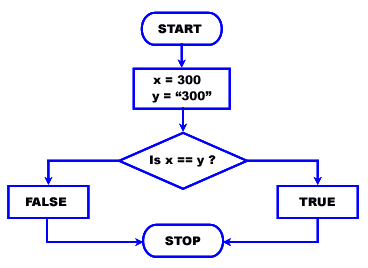Test Equal (==) operator

The following php codes return true though the type of \$x and \$y are not equal (the first one is integer type and the second one is character type) but their values are equal.

1. \$x = 300;

2. \$y = "300";

3. var_dump(\$x == \$y);

4. ?>

Output :

bool(true)

Pictorial presentation of Strict equal (===) operator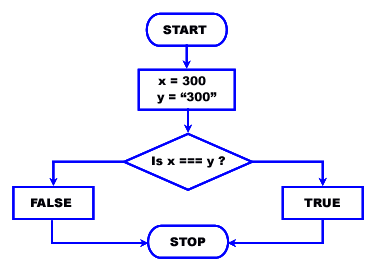Test Strict equal (===) operator

The following php codes returns false as the strict equal operator will compare both value and type of \$x and \$y.

1. \$x = 300;

2. \$y = "300";

3. var_dump(\$x === \$y);

4. ?>

Output :

bool(false)

View the example in the browser

Pictorial presentation of Not equal(!=)/(<>) operator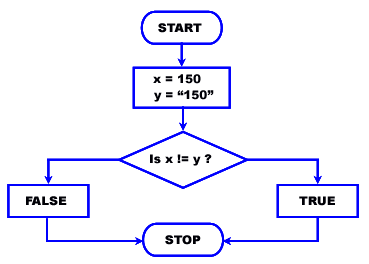Test Not equal (!=) / (<>) operator

The following php codes return false though the type of \$x and \$y are not equal (the first one is integer type and the second one is character type) but their values are equal.

view plaincopy to clipboardprint?

1.

2. \$x = 150;

3. \$y = "150";

4. var_dump(\$x != \$y);

5. ?>

Output of the example

bool(false)

View the example in the browser

Test Not identical (!==) operator

The following php codes return true though their values are equal but the type of \$x and \$y are not equal (the first one is integer type and the second one is character type).

1. \$x = 150;

2. \$y = "150";

3. var_dump(\$x !== \$y);

4. ?>

Output :

bool(true)

View the example in the browser

Pictorial presentation of Greater than(>) operator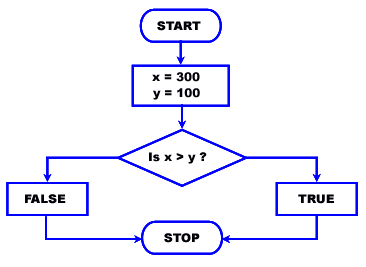Test Greater than(>) operator

The following php code return true as the value of \$x is greater than \$y.

1.

2. \$x = 300;

3. \$y = 100;

4. var_dump(\$x>\$y);

5. ?>

Output :

bool(true)

View the example in the browser

Pictorial presentation of Greater than or equal (>=)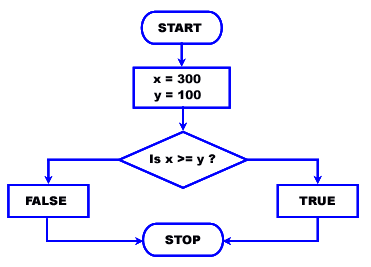Test Greater than or equal (>=) operator

The following php codes return true as the value of \$x is equal to \$y.

1. \$x = 300;

2. \$y = 100;

3. var_dump(\$x>=\$y);

4. ?>

Output :

bool(true)

View the example in the browser

Pictorial presentation of Less than (<) operator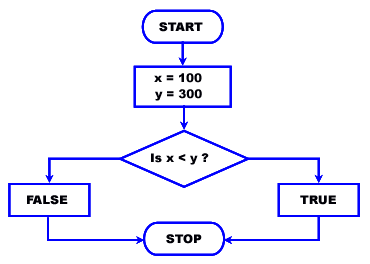Test Less than (<) operator

The following php codes return true as the value of \$x is less than \$y.

1. \$x = 100;

2. \$y = 300;

3. var_dump(\$x<\$y);

4. ?>

Output :

bool(true)

View the example in the browser

Pictorial presentation of Less than or equal (<=) operator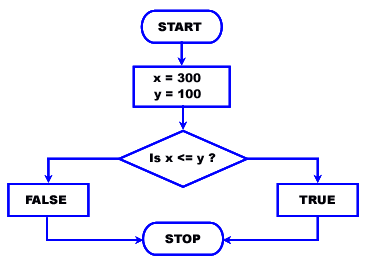Test Less than or equal (<=) operator

The following PHP codes return false as the value of \$x is greater than \$y.

view plaincopy to clipboardprint?

1. \$x = 300;

2. \$y = 100;

3. var_dump(\$x<=\$y);

4. ?>